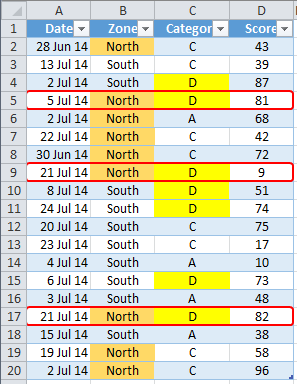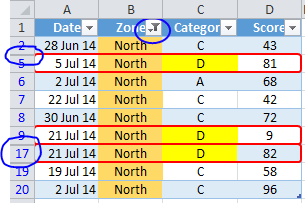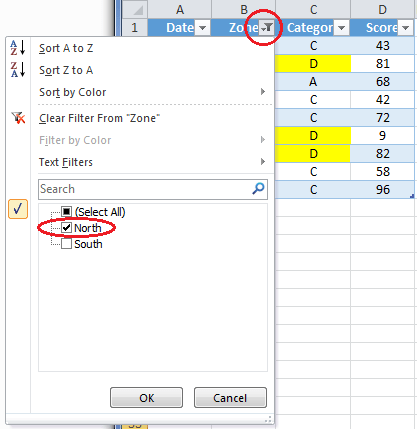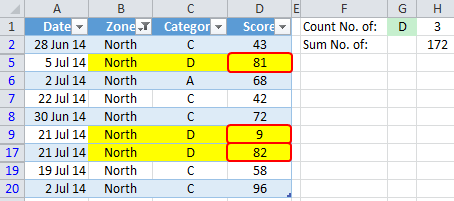# Formula Forensics No. 037 – How to Count and Sum Filtered Tables

A few weeks ago in the Chandoo.org Forums, Jsk_Lge asked “How do I count the number of items in a filtered list?

Narayan and I helped out with a well publicised Excel formula
=SUMPRODUCT(SUBTOTAL(103,OFFSET(A2,ROW(A2:A100)-ROW(A2),0)),(A2:A100=”D”)+0)

Today were going to unravel this and see what makes it tick.

## Formula

The Formula:
=SUMPRODUCT(SUBTOTAL(103,OFFSET(A2,ROW(A2:A100)-ROW(A2),0)),(A2:A100=”D”)+0) applied to Jsk’s data

We will use a Similar Formula better suited to our sample set of data

=SUMPRODUCT(SUBTOTAL(103,OFFSET(C1,ROW(A2:A20)-ROW(A1),0)),–(C2:C20=G1))We can see in the data above that there are 9 entries with a Zone of North (Orange), 7 entries with a Category of D (Yellow), including 3 Entries that have a Zone of North and a Category of D (Red)

If we filter the data so that Zone = North is selected, we will see there are three entries (Red) that match our criteria and that the three Scores sum to 172 (81+9+82).We can see the data is Filtered by the Blue color of the Row Labels, The larger Row Separator and Filter Icon at the Top of the Zone Column, all highlighted in Blue.

Jsk_Lge’s problem was how to Conditionally Count the Number of entries when the data is Filtered. eg: Category = D when the Zone is equal to North.

For this exercise please ensure the Data Table is filtered so that category North is selected## Solution

### Count Filtered Entries:

The solution is a Sumproduct based solution.

We know from Formula Forensics 007 that Sumproduct Sums the Product of the internal arrays.

In our formula: =SUMPRODUCT(SUBTOTAL(103,OFFSET(A1,ROW(A2:A20)-ROW(A1),0)),–(C2:C20=G1))
We can see there are two internal arrays
Array 1: =SUBTOTAL(103,OFFSET(A1,ROW(A2:A20)-ROW(A1),0))
Array 2: =–(C2:C20=”D”)

Lets look at each in turn:

Array 1: =SUBTOTAL(103,OFFSET(A1,ROW(A2:A20)-ROW(A1),0))

The Subtotal() returns a subtotal of a list or database. It has the functionality to work with Filtered Tables. The Syntax of the Subtotal() function is shown below:Specifically the Subtotal(103, Array) is designed to count the number of Visible entries in the array

But in our example the formula only appears to see an array involving Column A, it doesn’t look at our data column, Column C at all?
This first array is being used to specifically mark, in an array, which Rows are Visible = 1 or Hidden = 0

So what does the OFFSET(A1,ROW(A2:A20)-ROW(A1),0) part do?

The Offset() function is designed to return a range based on the criteria it is given

In this case it will return a range, which will be 1 cell high and 1 cell wide
It will be Offset from Cell A1 by a formula ROW(A2:A20)-ROW(A1) and in the same column as A1 (,0)

As the Offset formula is inside a Sumproduct Function it will be treated as an Array Formula.
This means that it will be processed for every value in the range ROW(A2:A20)

ie:
In Position 1 it will hold ROW(A2)-Row(A1) = 1
In Position 2 it will hold ROW(A3)-Row(A1) = 2
In Position 3 it will hold ROW(A4)-Row(A1) = 3
. . .
In Position 19 it will hold ROW(A20)-Row(A1) = 19

This will create a vertical array of 1..19 which can then be used by the Offset() function

The Offset Function will take this array of offset values and offsets A1 by each value in turn, in effect creating an Array of Ranges
ie:
In Position 1 it will Offset(A1, 1) = A2
In Position 2 it will Offset(A1, 2) = A3
In Position 3 it will Offset(A1, 3) = A4
. . .
In Position 19 it will Offset(A1, 19) = A5

So the Offset() function is returning an Array of Range Addresses to the Subtotal() function

Once again the Subtotal() function will be be treated as an array function as it is inside the Sumproduct() function.
This means that the Subtotal() function will be executed for every position in the Array

ie:
In Position 1 it will hold Subtotal(103, A2)
In Position 2 it will hold Subtotal(103, A3)
In Position 3 it will hold Subtotal(103, A4)
. . .
In Position 19 it will hold Subtotal(103, A20)

Now this is where the clever part kicks in!

The Subtotal(103, ) function will count the number of Visible values in the array
But as the array is an array of Single cell addresses, A2..A20

So:
if each value in A2..A20 is Visible it will be counted
if each value in A2..A20 is Hidden it won’t be counted

Lets check

In the sample file Filter Zone to show North Only

Goto cell H7 and press F2, then F9
Excel will return ={1;0;0;1;1;1;1;1;0;0;0;0;0;0;0;1;0;1;1}

This is showing a value of 1 for each Visible Row and a 0 for each hidden row

Try changing the filters and check the results

Array 2: =–(C2:C20=G1)

As we have seen in previous Formula Forensics, a simple formula like: –(C2:C20=G1) is a powerful way of adding criteria to a Formula

In this example –(C2:C20=G1) will return an Array of ={0;0;1;1;0;0;0;1;1;1;0;0;0;1;0;1;0;0;0}, but How

In the sample file goto cell H23 you will see a formula: =(C2:C20=G1)
Press F2 then F9
Excel returns: ={FALSE;FALSE;TRUE;TRUE;FALSE;FALSE;FALSE;TRUE;TRUE;TRUE;FALSE;FALSE;FALSE;TRUE;FALSE;TRUE;FALSE;FALSE;FALSE}

This is an array of True/False where each cell in range C2:C20 is compared to the cell G1
When they match it is True, When they don’t match it is False

The – – part is used to convert this array of True/False’s to an Array of 1/0’s
In the sample file cell H26 you will see a formula: =–(C2:C20=G1)
Press F2 then F9
Excel returns: ={0;0;1;1;0;0;0;1;1;1;0;0;0;1;0;1;0;0;0}

Finally We have our two arrays

=SUMPRODUCT(SUBTOTAL(103,OFFSET(A1,ROW(A2:A20)-ROW(A1),0)),–(C2:C20=G1))
which equates to :

=SUMPRODUCT({0;0;1;1;0;0;0;1;1;1;0;0;0;1;0;1;0;0;0} , {1;0;0;1;1;1;1;1;0;0;0;0;0;0;0;1;0;1;1} )

We can now see that Sumproduct will multiply the three arrays and add up the products
Array 1 {1;0;0;1;1;1;1;1;0;0;0;0;0;0;0;1;0;1;1}
Array 2 {0;0;1;1;0;0;0;1;1;1;0;0;0;1;0;1;0;0;0}
Array 1 x Array 2 {0;0;0;1;0;0;0;1;0;0;0;0;0;0;0;1;0;0;0}

Sumproduct correctly returns 3### Sum Filtered Entries:

Say we wanted to sum the values from the Score field based on Criteria and the Filtered data we can simply add another field to the original Sumproduct() function.

Eg: To sum the Score field whilst using the Criteria and Filtering we simply add a field to the end of the Sumproduct

=SUMPRODUCT(SUBTOTAL(103,OFFSET(A1,ROW(C2:C20)-ROW(A1),0)),(C2:C20=G1)*(D2:D20))

We can now see that Sumproduct will multiply the three arrays and add up the products
Array 1 = {1;0;0;1;1;1;1;1;0;0;0;0;0;0;0;1;0;1;1}
Array 2 = {0;0;1;1;0;0;0;1;1;1;0;0;0;1;0;1;0;0;0}
Array 3 = {43;39;87;81;68;42;72;9;51;74;75;17;10;73;48;82;38;58;96}
Array 1 x Array 2 x Array 3 = {0;0;0;81;0;0;0;9;0;0;0;0;0;0;0;82;0;0;0}

Sumproduct correctly returns 172

## A Challenge

Can you solve the Count problem another way ?

Can you solve the Sum problem another way ?

## Other Posts in this Series

The Formula Forensics Series contains a wealth of useful solutions and information specifically about how Array Formula work.Hello Awesome...

My name is Chandoo. Thanks for dropping by. My mission is to make you awesome in Excel & your work. I live in Wellington, New Zealand. When I am not F9ing my formulas, I cycle, cook or play lego with my kids. Know more about me.

Thank you and see you around.

### Related articles:

 Written by Hui... Tags: row(), SUBTOTAL, sumproduct Home: Chandoo.org Main Page ? Doubt: Ask an Excel Question

### 23 Responses to “Formula Forensics No. 037 – How to Count and Sum Filtered Tables”

1.Sumit Bansal says:

Nice trick there with Subtotal

2.Rob T says:

Here's a "helper column" method, which uses the same idea. It also avoids using OFFSET, which is volatile:

(I know we should avoid helper columns where possible, but this is easier to understand and recreate from memory, without referring back to this blog post)

Add a column E to the table with the following formula in E2 and copied down:
=SUBTOTAL(103,\$A2)
This will return 1 for visible rows and 0 for hidden rows.

Then, the formula for counting the number of visible D's becomes:
=COUNTIFS(C2:C20,"D",E2:E20,1)

And to sum the Scores for visible D's:
=SUMIFS(D2:D20,C2:C20,"D",E2:E20,1)

•Hui... says:

@Rob

Although Offset is Volatile, a few or even a few dozen Offset's will not hurt the performance of a worksheet.
It is when people use them to make large tables of thousands of cells that the performance degrades.

•Rob T says:

Hui,
I appreciate that in this case OFFSET doesn't impact performance. The main benefit really is that I'll struggle to remember and recreate the "better" solution above!

3.Utkarsh Shah says:

Hi Chandoo,

I think simple solution for Count & Sum problem is use subtotal formula with 2 & 9 number instead 102 & 109 number for count & sum function resp.
e.g.
=SUBTOTAL(2,A4:A8) - For Count of range A4 to A8

=SUBTOTAL(9,A4:A8) - For Sum of range A4 to A8

When you apply filters, sum & count will autochanges.

If you want to sum & count all entries (Hidden & visible) then use 102 & 109.

•Hui... says:

@Utkarsh
Your formula's work for filtered data, but they don't allow for additional criteria to be applied as well as the filtering
ie: In the examples shown To Count or Sum the entries that have a category Value of D whilst being filtered for the Zone = North values

•Rob says:

I agree with Utkarsh Shah. If you filter you can have multiple criteria applied one for each column, which gets you down to teh three records you want. Then Then the subtotal will count them in one column and you can also have a sum in the score column. Or add any of a dozen different aggregation or info pulling formulas there. They all adjust based on the filters and we are all happy. Unless a formula answer was needed to put the number somewhere else; then just refer to the answer cells.

•Hui... says:

@Rob
But in cases where you want to see what proportion of say the Filtered Zone=North records was Category = D, you need to have the Data only partially filtered at the Zone level.

4.Darin Myers says:

I know this is a formula breakdown, but my approach would be to use a simple pivot table.

Many here are pros, so I won't into detail about how to handle these calculations in a pivot table, all I will say is that I can't count how many times people have been thankful to me for pointing them to a better method after helping them figure out the method they were planning on using.

5.Jomili says:

I'm confused. Why wouldn't you simply use Countifs and Sumifs?
=COUNTIFS(\$C\$2:\$C\$20,"D",\$B\$2:\$B\$20,"North")
=SUMIFS(D2:D20,\$C\$2:\$C\$20,"D",\$B\$2:\$B\$20,"North")

•Colleen says:

I'm with you, Jomili. I always use COUNTIFS and SUMIFS. Why wouldn't they work? It seems much simpler.

Unless maybe they are using a version older than Excel 2007? If I recall, these functions were introduced in Excel 2007, making my happiness meter improve by leaps and bounds.

6.Grant says:

Perhaps I am missing something but why not use DSUM and DCOUNT?

The range is already a table.
I put the Criteria of Zone and Category in a new range (F37:G38) and did the following:

=DSUM(Table1[#All], D1, F37:G38) = 172
=DCOUNT(Table1[#All], D1, F37:G38) = 3

D1 - is the cell that has Score.

Get same answers irrespective of whether the table is filtered or not.

7.Marci says:

I would also use one of three approaches depending on how often I would need to use the info:

Pivot table
Sumifs and Countifs formulae
Subtotal 9 and Subtotal 2 on a row below the entire table

8.Haz says:

=SUMPRODUCT((Table1[Zone]="North")+0, (Table1[Category]="D")+0) = 3

=SUMPRODUCT((Table1[Zone]="North")+0, (Table1[Category]="D")+0, Table1[Score]) = 172

9.David Clapperton says:

Standing on Mike Girvin's shoulders, rather that filter the list I would be tempted to have two cells, each data validated to select a Zone (say A22) and a category (say A23). Then use these as lookups to feed into a three way array multiplication formula for the sum =SUMPRODUCT(- -(B2:B20=G22),- -(C2:C20=G23),D2:D20) and a two way for the count =SUMPRODUCT(- -(B2:B20=G22),- -(C2:C20=G23)) . These use the clever double negation trick to convert True/False into 1/0 before multiplying out. IMHO - a little easier to follow the logic as well.

•David Clapperton says:

Sorry, Double negatives before each of the terms - didn't come out in the conversion.

10.Asghar Abbasnejad says:

The short solve are:
=COUNTIFS(\$C\$2:\$C\$20,"D",\$B\$2:\$B\$20,"North")
=SUMIFS(D2:D20,\$C\$2:\$C\$20,"D",\$B\$2:\$B\$20,"North")

With best regards

11.Jonathan James says:

I feel the point of this article was to design a formula that would update when data is filtered, and that wouldnt require the criteria to be specified elsewhere in the sheet. That being said, I dont have an alternative to one discussed in the article above.

When I'm using SUMPRODUCT, I prefer to multiply the ranges rather use the double negation trick ( - - ) to convert TRUE,FALSE to 1,0.

This formula: =SUMPRODUCT(- -(B2:B20=G22),- -(C2:C20=G23),D2:D20)
becomes: =SUMPRODUCT((B2:B20=G22)*(C2:C20=G23),D2:D20)

•John Jairo V says:

Hi Jonathan.

Although time has passed , I want to clarify that the syntax with the double negative is more efficient than the multiplication between two ranges. That's why in short is better to use double negative. Blessings !

12.Jeff Weir says:

I've somehow missed this until now. That SUBTOTAL/OFFSET combination is killer.

aMaeris uses a similar SUBTOTAL/OFFSET trick at http://excelxor.com/2015/07/20/advanced-formula-challenge-12-an-array-of-matches/#comment-1366

And I see there's a good writeup over at http://dailydoseofexcel.com/archives/2005/05/11/arrays-with-offset/

13.CookieRevised says:

This week I had a similar challenge. It is a kind of variation on the problem explained above:

How to count the number of unique text values in a column...
1) WITHOUT the use of any helper-column!!!
2) taking in account possible empty cells
3) taking in account filtering on that and other columns
4) taking in account possible hidden rows
5) (without the use of VBA of course... would be too easy otherwise :-P)

Solutions found on the web (when you search hard enough):
- almost always involve a helper-column
- or do not calculate correctly if there is no filtering (it is 1 off in that case, because of empty rows which are included in the count)
- or only work on number values at best, not text values...
- and almost always involve the construct SUMPRODUCT(SUBTOTAL(103,OFFSET()),SOME_REF_TO_A_FIXED_LOOKUP_CELL). This makes that this would always need helper columns or at least one helper cell. This is useless if you only want to check unique values in the column.

Another problem with this is that Excel does not like to take a SUBTOTAL() of a SUBTOTAL(). Result is that you actually need two helper columns for this if you want to use the SUMPRODUCT(SUBTOTAL()) construct.

Exmaple and solution:

You have a column with city names, and you want to count the total number of unique cities in that column. But that total number of unique cities should also update when columns are filtered (say all cities starting with A*).

A1: the text header, with autofiltering on or off
A2 thru A10: the citie names

The matrix formula to count and sum the values:
=COUNT(
IF(LEN(A2:A20);
IF(INTERVAL(
IF(SUBTOTAL(103;OFFSET(A2:A20;ROW(A2:A20)-ROW(A2);;1));MATCH(A2:A20;A2:A20;0));
IF(SUBTOTAL(103;OFFSET(A2:A20;ROW(A2:A20)-ROW(A2);;1));MATCH(A2:A20;A2:A20;0))
)
;1)
)
)

note: this is a matrix formula, so enter it using CTRL-SHIFT-ENTER
note: you can replace COUNT with SUM
note: COUNTIF and SUMIF will not work

•CookieRevised says:

Sorry, above formula has a bug in it when you also sort columns after filtering..... and I forgot to translate the FREQUENCY function to English, together with the proper used delimiters in English version of Excel...

oopsie...

The proper matrix formula is:
=COUNT(
IF(
FREQUENCY(
IF(LEN(A2:A20),IF(SUBTOTAL(3,OFFSET(A2:A20,ROW(A2:A20)-ROW(A2),,1)),MATCH(A2:A20,A2:A20,0))),
IF(LEN(A2:A20),IF(SUBTOTAL(3,OFFSET(A2:A20,ROW(A2:A20)-ROW(A2),,1)),MATCH(A2:A20,A2:A20,0)))
)
,1)
)

14.Chirag Raval says:

Dear Sirs & Users,

This Is Really mind blowing concept that to learn how to receive various required effect on fix (waiting for result) structure based on dynamically changed database.

But , base on overview of this concept, I can point towards that all last row reference is hard coded.

Like (A1:A550) can it accept last row as dynamically (auto initialised last row address) like (A1:A & lastRow)?

Require this due to for if we want to use this method on regularly/daily/ hourly basis on received every dynamic data range.

Hope there are some solution there.

Regards,

Chirag Raval

 « Ask me your Excel questions & You could win an eBook CP015: Handling big data, Controlling model railroad sets, Overcoming Excel obsession & more – ASK CHANDOO »

### Get FREE Excel & Power-BI Newsletter

One email per week with Excel and Power BI goodness. Join 100,000+ others and get it free.